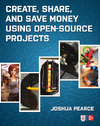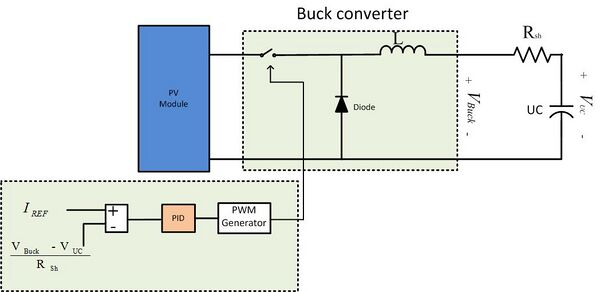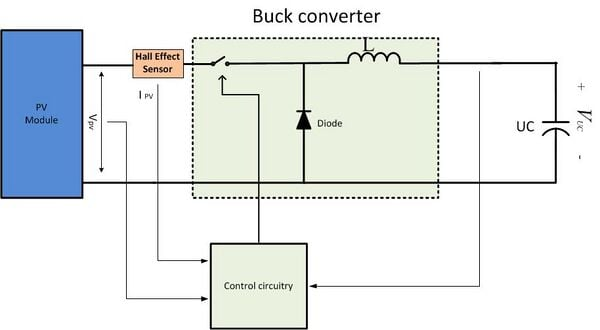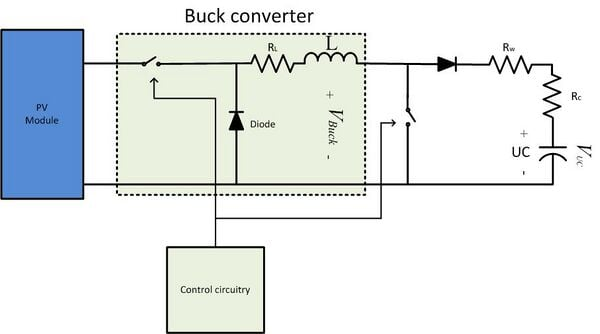Michigan Tech's Open Sustainability Technology Lab. Contact Dr. Joshua Pearce now at Free Appropriate Sustainable Technology MOST: Projects & Publications, Methods, Lit. reviews, People, Sponsors, News Updates: Twitter, YouTubeThis project describes the power electronics necessary for charging a bank of ultracapitors with PV

# Ultracapacitor charging topologies:

The Ultra capacitors can be charged mainly using three topologies:

### Constant Current Charging (CCCM)

 A DC-DC buck converter is used to charge the ultra capacitor bank. In the CCCM a constant current is used to charge the bank, the constant current can be monitored by using a series resistance of low value. The voltage across the resistor is measured, and is kept constant by varying the duty ratio of the buck converter, usually by making use of a DSP controller or by using a microcontroller.Figure 1. Constant current charging mode schematic As shown in the Figure 1 we can see that in the control circuitry the current through the series resistance is taken as the input (voltage across the resistance /Resistance). This is compared to a constant reference value of current we want to charge the capacitor bank. The PID controller then decides the operating duty ratio of the buck converter. The CCCM is advantageous when the capacitor bank is at zero voltage, in this situation, if a constant voltage were applied to the terminals of the bank, a large surge of current is drawn in, damaging the on board electronics.

### Constant power charging mode (CPCM)

A DC-DC converter (preferably a buck converter is made use of) in the CPCM. In this mode a constant charging power P is assumed depending on power output of the solar PV module, the input voltage and the input current are measured and then by varying the duty ratio of the buck converter the current drawn is controlled, thereby maintaining the power at a the constant level of P.Figure 2. Constant Power charging mode schematic. Similar to the CCCM the duty ratio is varied to control the input current and voltage, this is usually done by using a DSP controller or a microcontroller. The CPCM is advantageous when the capacitor bank is not at zero voltage and the charging time is a critical issue, this type of charging is usually advantageous in regenerative braking where the, energy generated is for a very small amount of time.

### Discontinuous charging

 Discontinuous charging is different from the above two methods, in this method there is no constant current flowing into the capacitor bank. A small inductance is taken, and the circuit is a modification of the Buck converter. Two synchronous Mosfets are used in this circuit. When the Mosfets are turned on the, inductor is charged, and when they are turned off, the inductor is discharged through the two freewheeling diodes to the capacitor bank.Figure 3. Discontinuous charging schematic.

The advantages of this circuit is that the circuit does not require any closed circuit feedback mechanism, and it does not involve any large inductance. But the two freewheeling diodes are the biggest current limiting components in the circuit and a lot of energy is dissipated in the diodes, which might be a problem.